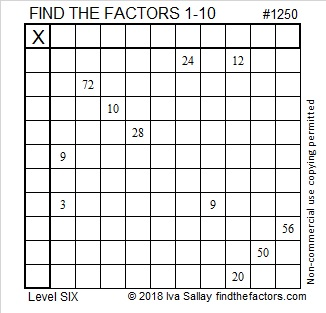# 1250 and Level 6

The clues in one of the columns for this puzzle as well as one of the rows are 9 and 3. You will need to figure out where to put the factors 1, 3, 3, and 9 to make those clues work. You might think it doesn’t matter where you write those factors, but believe me, it does matter. My advice: Don’t start with those clues. Find another logical place to start. Good luck with this one!Print the puzzles or type the solution in this excel file: 10-factors-1242-1250

Here are some facts about the number 1250:

• 1250 is a composite number.
• Prime factorization: 1250 = 2 × 5 × 5 × 5 × 5, which can be written 1250 = 2 × 5⁴
• The exponents in the prime factorization are 1 and 5. Adding one to each and multiplying we get (1 + 1)(4 + 1) = 2 × 5 = 10. Therefore 1250 has exactly 10 factors.
• Factors of 1250: 1, 2, 5, 10, 25, 50, 125, 250, 625, 1250
• Factor pairs: 1250 = 1 × 1250, 2 × 625, 5 × 250, 10 × 125, or 25 × 50
• Taking the factor pair with the largest square number factor, we get √1250 = (√625)(√2) = 25√2 ≈ 35.355341250 is the sum of consecutive squares two different ways:
193 + 197 + 199 + 211 + 223 + 227 = 1250
619 + 631 = 1250

1250 is the sum of two squares THREE different ways:
31² + 17² = 1250
25² + 25² = 1250
35² + 5² = 1250

1250 is the hypotenuse of FOUR Pythagorean triples:
750-1000-1250 which is (3-4-5) times 250,
672-1054-1250 which is 2 times (336-527-625) and
can also be calculated from 31² – 17², 2(31)(17), 31² + 17²,
440-1170-1250 which is 10 times (44-117-125), and
350-1200-1250 which is (7-24-25) times 50 and
can also be calculated from 2(35)(5), 35² – 5², 35² + 5²

This site uses Akismet to reduce spam. Learn how your comment data is processed.